# HSPT Math : Concepts

## Example Questions

### Example Question #81 : Arithmetic

Fill in the blank with the proper sign.__________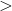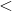Explanation:

In order to solve this problem, we will use a number line. Numbers to the left of zero on the line are less than numbers to the right.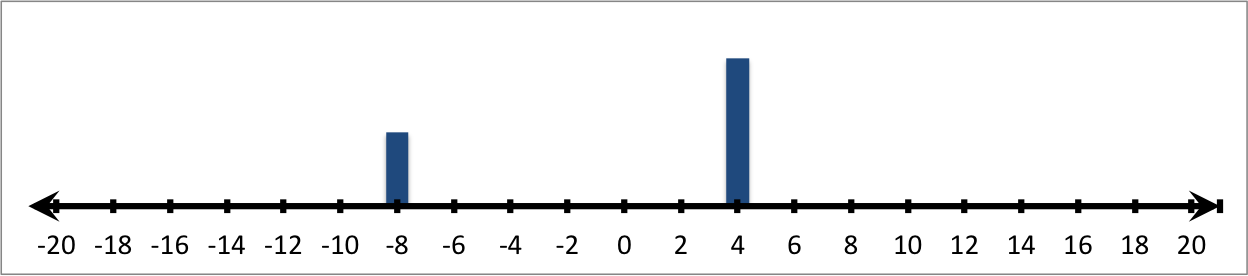Therefore: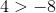### Example Question #491 : The Number System

Fill in the blank with the proper sign.__________Explanation:

In order to solve this problem, we will use a number line. Numbers to the left of zero on the line are less than numbers to the right.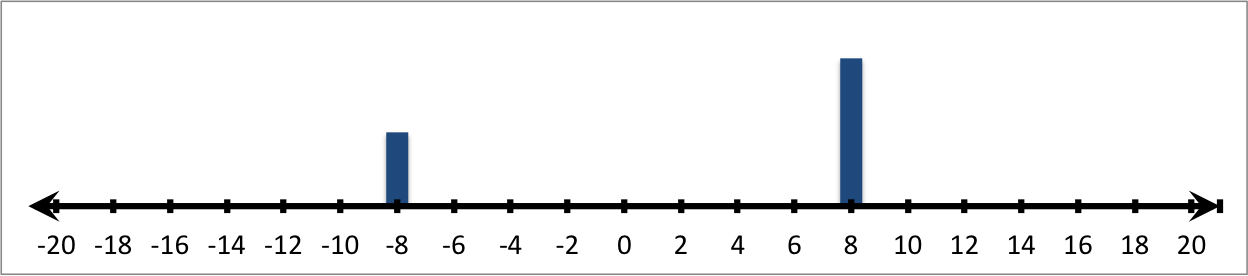Therefore: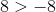### Example Question #41 : Understand An Inequality On A Number Line: Ccss.Math.Content.6.Ns.C.7a

Fill in the blank with the proper sign.__________Explanation:

In order to solve this problem, we will use a number line. Numbers to the left of zero on the line are less than numbers to the right.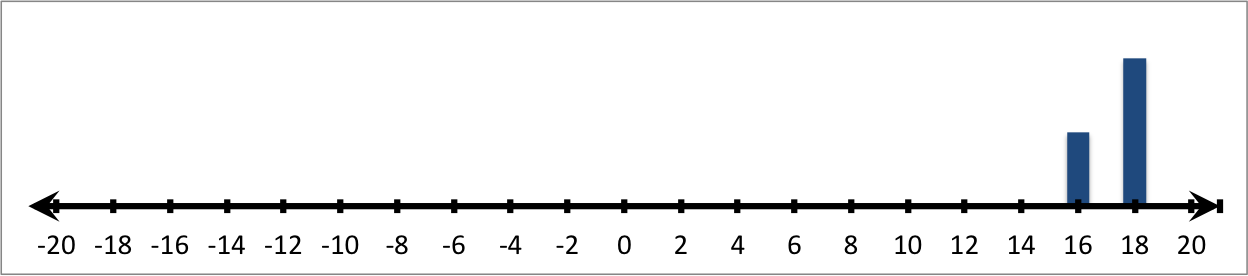Therefore: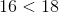### Example Question #42 : Understand An Inequality On A Number Line: Ccss.Math.Content.6.Ns.C.7a

Fill in the blank with the proper sign.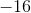__________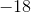Explanation:

In order to solve this problem, we will use a number line. Numbers to the left of zero on the line are less than numbers to the right.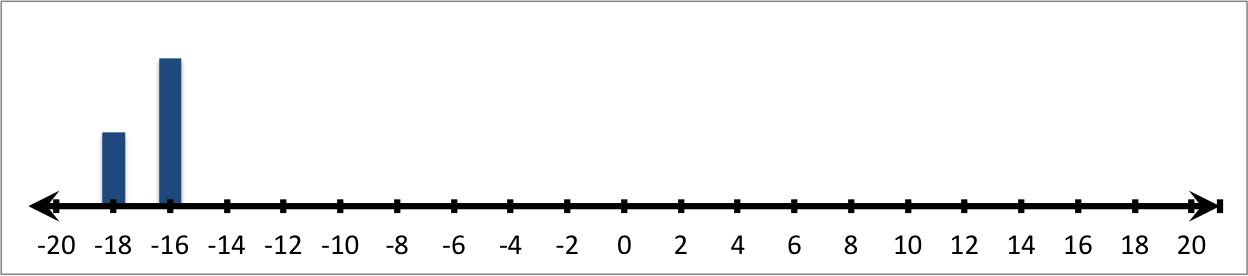Therefore: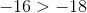### Example Question #581 : Grade 6

Fill in the blank with the proper sign.__________Explanation:

In order to solve this problem, we will use a number line. Numbers to the left of zero on the line are less than numbers to the right.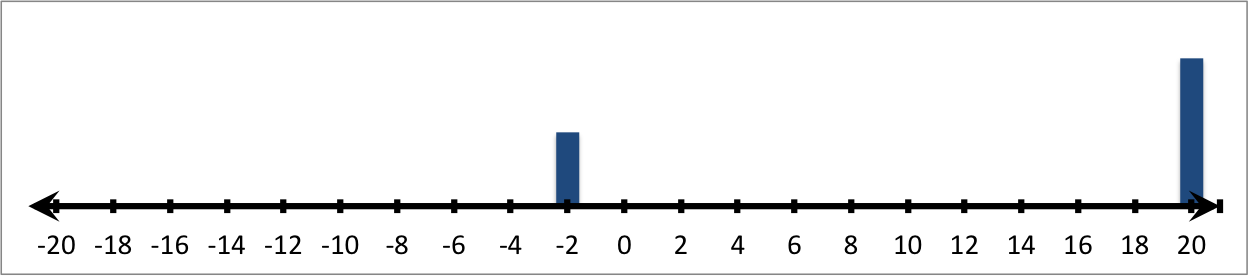Therefore: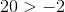### Example Question #494 : The Number System

Fill in the blank with the proper sign.__________Explanation:

In order to solve this problem, we will use a number line. Numbers to the left of zero on the line are less than numbers to the right.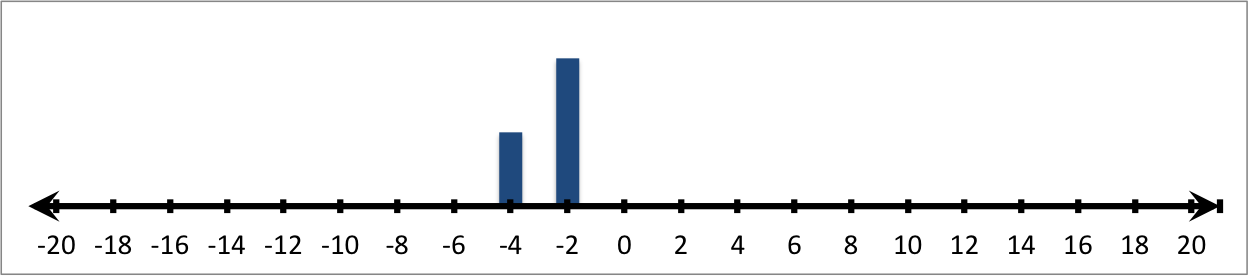Therefore: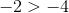### Example Question #43 : Understand An Inequality On A Number Line: Ccss.Math.Content.6.Ns.C.7a

Fill in the blank with the proper sign.__________Explanation:

In order to solve this problem, we will use a number line. Numbers to the left of zero on the line are less than numbers to the right.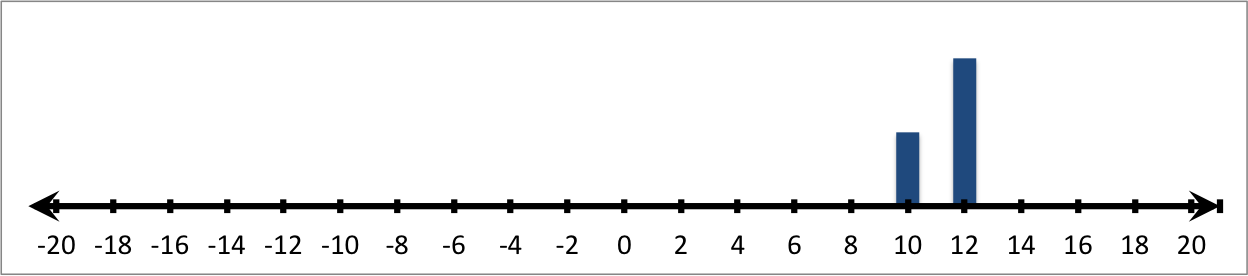Therefore: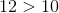### Example Question #44 : Understand An Inequality On A Number Line: Ccss.Math.Content.6.Ns.C.7a

Fill in the blank with the proper sign.__________Explanation:

In order to solve this problem, we will use a number line. Numbers to the left of zero on the line are less than numbers to the right.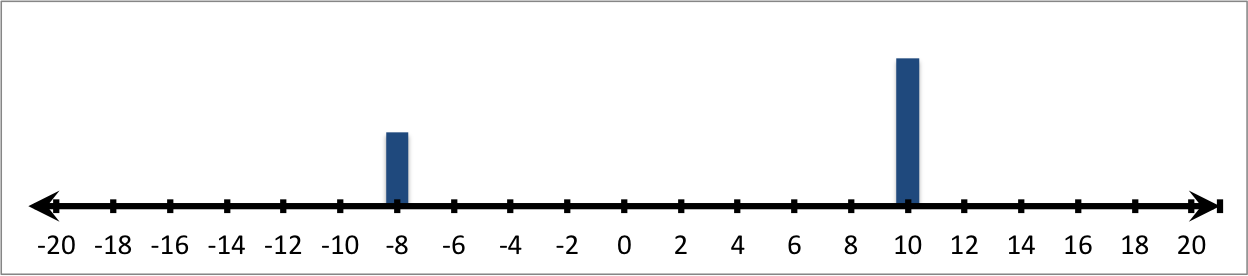Therefore: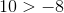### Example Question #581 : Grade 6

Fill in the blank with the proper sign.__________Explanation:

In order to solve this problem, we will use a number line. Numbers to the left of zero on the line are less than numbers to the right.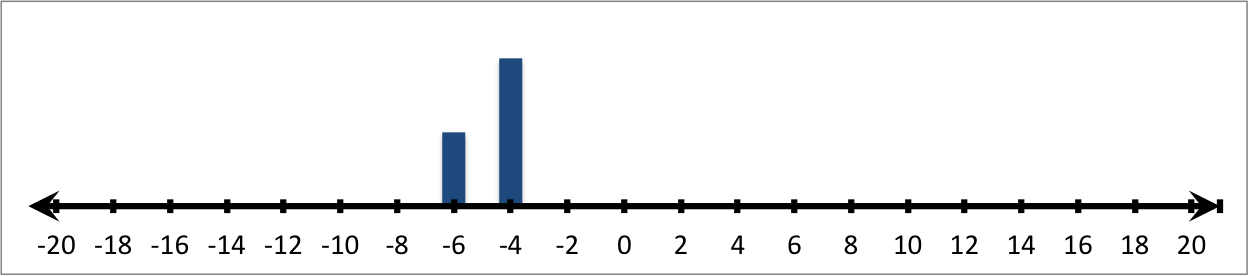Therefore: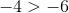### Example Question #81 : Negative Numbers

Adding a negative number to a positive number will ALWAYS be negative. True or false?

True

False

False

Explanation:

If the positive number is a larger constant than the negative number, your final answer will be positive so the statement above is incorrect.

Example: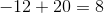### All HSPT Math Resources